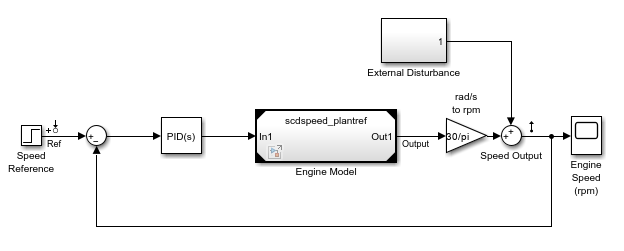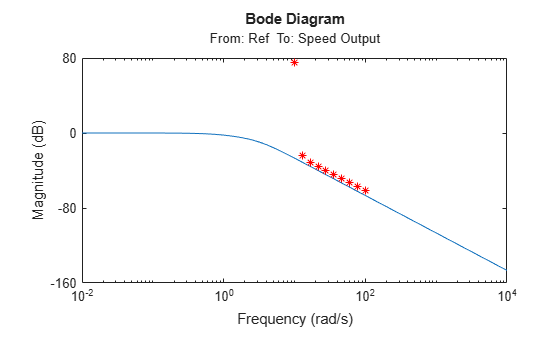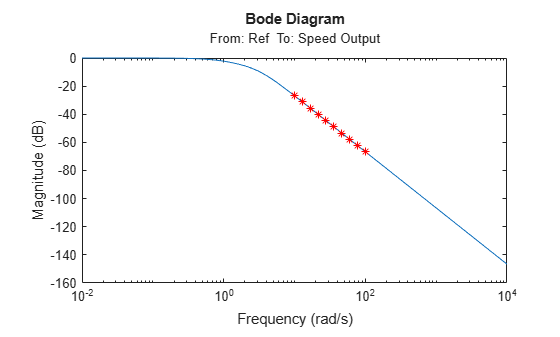# frest.findSources

Package: frest

Identify time-varying source blocks

## Syntax

``blocks = frest.findSources(model)``
``blocks = frest.findSources(model,io)``

## Description

Time-varying source blocks in a Simulink® model can interfere with frequency response estimation by driving the model away from the operating point of the linearized system. Using the `frest.findSources` function, you can identify such time-varying sources in your model. You can then disable the identified sources before estimating a frequency response using `frestimate`. For more information, see Effects of Time-Varying Source Blocks on Frequency Response Estimation.

````blocks = frest.findSources(model)` finds all time-varying source blocks in the signal path of any linearization output point marked in the Simulink model `model`.```

example

````blocks = frest.findSources(model,io)` finds all time-varying source blocks in the signal path of any linearization output point specified in the array of linear analysis points `io`.```

## Examples

collapse all

Open a model that contains time-varying source blocks.

```mdl = "scdspeed_ctrlloop"; open_system(mdl)```Set the engine reference model to normal simulation mode for accurate linearization.

```set_param("scdspeed_ctrlloop/Engine Model",... "SimulationMode","Normal")```

Obtain the linear analysis points that are defined in the model.

`io = getlinio(mdl);`

Estimate the frequency response of the model without disabling the time-varying source blocks. Define a sinestream input signal and obtain the estimated frequency response `sysest`.

```in = frest.Sinestream(... "Frequency",logspace(1,2,10),... "NumPeriods",30,... "SettlingPeriods",25); [sysest,simout] = frestimate(mdl,io,in);```

To view the impact of the time-varying sources on the estimation result, compute an exact linearization of the model and compare it to the estimated response.

```sys = linearize(mdl,io); bodemag(sys,sysest,'r*')```The estimated frequency response does not match the exact linearization. The mismatch occurs because time-varying source blocks in the model prevent the response from reaching steady state.

Find the time-varying source blocks.

`srcblks = frest.findSources(mdl); `

To disable the source blocks, first create an `frestimateOptions` object and set the `BlocksToHoldConstant` parameter to the time-varying source blocks.

`opts = frestimateOptions("BlocksToHoldConstant",srcblks);`

Estimate the frequency response with the time-varying source blocks disabled.

`[sysest2,simout2] = frestimate(mdl,io,in,opts);`

Compare the estimated response `sysest2` with the exact linearization result.

`bodemag(sys,sysest2,'r*')`The estimated response matches the exact linearization.

## Input Arguments

collapse all

Name of the Simulink model for which you want to identify time-varying sources.

Linear analysis points representing inputs, outputs, and loop openings, specified as a linearization I/O object or an array of such objects. The `frest.findSources` function uses only the linearization output points in `io`.

For more information on specifying linear analysis points, see Specify Portion of Model to Linearize and Specify Portion of Model to Linearize at Command Line.

## Output Arguments

collapse all

Time-varying source blocks, returned as a array of `BlockPath` objects. `blocks` includes time-varying source blocks inside subsystems and normal-mode referenced models.

If you specify `io`, `blocks` contains all time-varying source blocks contributing to the signal at the output analysis points in `io`.

If you do not specify `io`, `blocks` contains all time-varying source blocks contributing to the signals at the output analysis points marked in `model`. For more information on specifying analysis points in your model, see Specify Portion of Model to Linearize in Simulink Model.

## Tips

• To disable time-varying source blocks during frequency response estimation, first create an `frestimateOptions` object and set its `BlocksToHoldConstant` property to `blocks` or a subset of `blocks`. Then, estimate the frequency response using `frestimate`.

• When `model` includes a reference model that contains a source block in the signal path of a linearization output point, set the reference model to normal simulation mode before finding sources using `frest.findSources`.